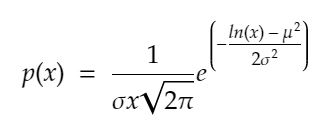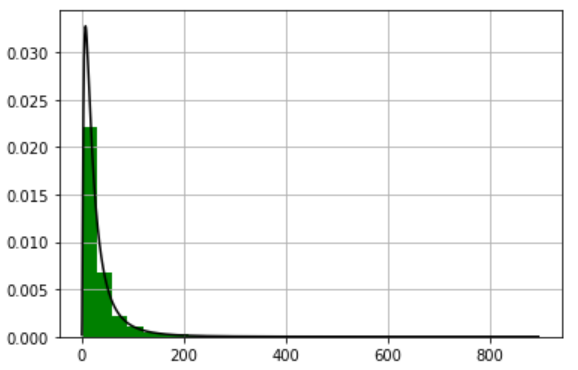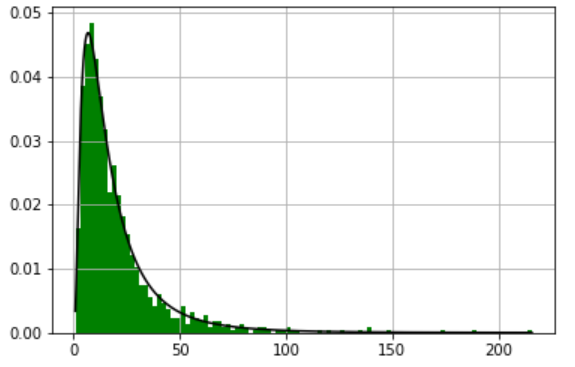Open in App
Not now

# How to generate random numbers from a log-normal distribution in Python ?

• Last Updated : 07 Apr, 2021

A continuous probability distribution of a random variable whose logarithm is usually distributed is known as a log-normal (or lognormal) distribution in probability theory.

A variable x is said to follow a log-normal distribution if and only if the log(x) follows a normal distribution. The PDF is defined as follows.Probability Density function Log-normal

Where mu is the population mean & sigma is the standard deviation of the log-normal distribution of a variable. Just like normal distribution which is a manifestation of summation of a large number of Independent and identically distributed random variables, lognormal is the result of multiplying a large number of Independent and identically distributed random variables. Generating a random number from a log-normal distribution is very easy with help of the NumPy library.

Syntax:

numpy.random.lognormal(mean=0.0, sigma=1.0, size=None)

Parameter:

• mean: It takes the mean value for the underlying normal distribution.
• sigma: It takes only non-negative values for the standard deviation for the underlying normal distribution
• size : It takes either a int or a tuple of given shape. If a single value is passed it returns a single integer as result. If a tuple then it returns a 2D matrix of values from log-normal distribution.

Returns: Drawn samples from the parameterized log-normal distribution(nd Array or a scalar).

The below example depicts how to generate random numbers from a log-normal distribution:

## Python3

 `# import modules``import` `numpy as np``import` `matplotlib.pyplot as plt`` ` `# mean and standard deviation``mu, sigma ``=` `3.``, ``1.`  `s ``=` `np.random.lognormal(mu, sigma, ``10000``)`` ` `# depict illustration``count, bins, ignored ``=` `plt.hist(s, ``30``,``                                ``density``=``True``, ``                                ``color``=``'green'``)``x ``=` `np.linspace(``min``(bins),``                ``max``(bins), ``10000``)`` ` `pdf ``=` `(np.exp(``-``(np.log(x) ``-` `mu)``*``*``2` `/` `(``2` `*` `sigma``*``*``2``))``       ``/` `(x ``*` `sigma ``*` `np.sqrt(``2` `*` `np.pi)))`` ` `# assign other attributes``plt.plot(x, pdf, color``=``'black'``)``plt.grid()``plt.show()`

Output:Let’s prove that log-Normal is a product of independent and identical distributions of a random variable using python. In the program below we are generating 1000 points randomly from a normal distribution and then taking the product of them and finally plotting it to get a log-normal distribution.

## Python3

 `# Importing required modules``import` `numpy as np``import` `matplotlib.pyplot as plt`` ` `b ``=` `[]`` ` `# Generating 1000 points from normal distribution.``for` `i ``in` `range``(``1000``):``    ``a ``=` `12.` `+` `np.random.standard_normal(``100``)``    ``b.append(np.product(a))`` ` `# Making all negative values  into positives``b ``=` `np.array(b) ``/` `np.``min``(b)``count, bins, ignored ``=` `plt.hist(b, ``100``, ``                                ``density``=``True``, ``                                ``color``=``'green'``)`` ` `sigma ``=` `np.std(np.log(b))``mu ``=` `np.mean(np.log(b))`` ` `# Plotting the graph.``x ``=` `np.linspace(``min``(bins), ``max``(bins), ``10000``)``pdf ``=` `(np.exp(``-``(np.log(x) ``-` `mu)``*``*``2` `/` `(``2` `*` `sigma``*``*``2``))``       ``/` `(x ``*` `sigma ``*` `np.sqrt(``2` `*` `np.pi)))`` ` `plt.plot(x, pdf,color``=``'black'``)``plt.grid()``plt.show()`

Output:My Personal Notes arrow_drop_up